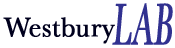Data

CorrelationPCalc: A tool for calculating p value of a linear correlation

Description: This Excel spreadsheet will calculate the p-value of a linear correlation, given an N and r. With a little effort (using Excel’s ‘Seek goal’ tool), you can also use it tell you what r value you would need with a given population to get a specific significance level. The spreadsheet uses the fact that sqrt[(1—r2)/(N—2)] is distributed approximately as t with df=N—2.

Citation:  Westbury, C. (2008) CorrelationPCalc: A tool for calculating p value of a linear correlation  Edmonton, AB: University of Alberta (downloaded from http://www.psych.ualberta.ca/~westburylab/downloads/corrpcalc.html)

Acknowledgments: This research was supported by NSERC.Please fill out this form so that we can keep track of who has downloaded this file.

Full Name: